# 常见算法总结

• 面试手册：这是一份大彬精心整理的大厂面试手册open in new window最新版，目前已经更新迭代了19个版本，质量很高（专为面试打造）
• 知识星球专属面试手册/一对一交流/简历修改/超棒的学习氛围/学习路线规划，欢迎加入大彬的知识星球open in new window（点击链接查看星球的详细介绍）

## # 二叉树的遍历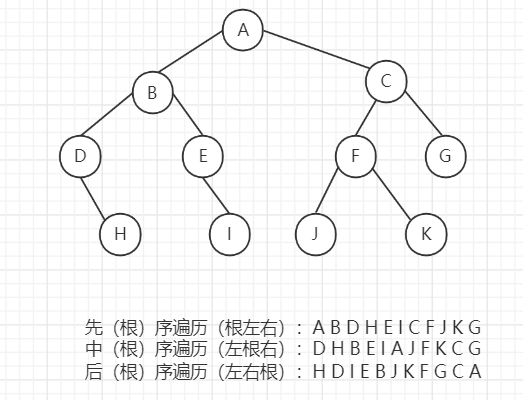### # 前序遍历

1. 访问结点，并把结点node入栈，当前结点置为左孩子；
2. 判断结点node是否为空，若为空，则取出栈顶结点并出栈，将右孩子置为当前结点；否则重复a)步直到当前结点为空或者栈为空（可以发现栈中的结点就是为了访问右孩子才存储的）
``````
public void preOrderTraverse2(TreeNode root) {
TreeNode pNode = root;
while (pNode != null || !stack.isEmpty()) {
if (pNode != null) {
System.out.print(pNode.val+"  ");
stack.push(pNode);
pNode = pNode.left;
} else { //pNode == null && !stack.isEmpty()
TreeNode node = stack.pop();
pNode = node.right;
}
}
}
``````

### # 中序遍历

``````    public List<Integer> inorderTraversal(TreeNode root) {
List<Integer> res = new ArrayList<>();
Deque<TreeNode> deque = new ArrayDeque<>();

while (!deque.isEmpty() || root != null) {
while (root != null) {
deque.push(root);
root = root.left;
}
root = deque.pop();
root = root.right;
}

return res;
}
``````

### # 后序遍历

``````class Solution {
public List<Integer> postorderTraversal(TreeNode root) {
if (root == null) {
return res;
}

Stack<TreeNode> stack = new Stack<>();
stack.push(root);
while (!stack.isEmpty()) {
root = stack.pop();
if (root != null) {
stack.push(root);//最后访问
stack.push(null);
if (root.right != null) {
stack.push(root.right);
}
if (root.left != null) {
stack.push(root.left);
}
} else { //值为null说明此次递归调用结束，将节点值存进结果
}
}

return res;
}
}
``````

### # 层序遍历

``````public void levelTraverse(TreeNode root) {
if (root == null) {
return;
}
queue.offer(root);
while (!queue.isEmpty()) {
TreeNode node = queue.poll();
System.out.print(node.val+"  ");
if (node.left != null) {
queue.offer(node.left);
}
if (node.right != null) {
queue.offer(node.right);
}
}
}
``````

## # 排序算法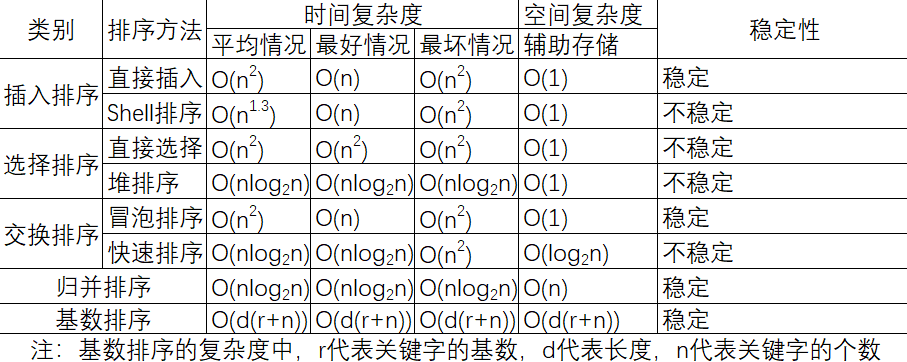### # 冒泡排序

• 比较相邻的元素。如果第一个比第二个大，就交换它们两个；
• 对每一对相邻元素作同样的工作，从开始第一对到结尾的最后一对，这样在最后的元素应该会是最大的数；
• 针对所有的元素重复以上的步骤，除了最后一个；
• 重复步骤1~3，直到排序完成。

``````public void bubbleSort(int[] arr) {
if (arr == null) {
return;
}
boolean flag;
for (int i = arr.length - 1; i > 0; i--) {
flag = false;
for (int j = 0; j < i; j++) {
if (arr[j] > arr[j + 1]) {
int tmp = arr[j];
arr[j] = arr[j + 1];
arr[j + 1] = tmp;
flag = true;
}
}
if (!flag) {
return;
}
}
}
``````

### # 插入排序

• 从第一个元素开始，该元素可以认为已经被排序；
• 取出下一个元素，在已经排序的元素序列中从后向前扫描；
• 如果该元素（已排序）大于新元素，将该元素移到下一位置；
• 重复步骤3，直到找到已排序的元素小于或者等于新元素的位置；
• 将新元素插入到该位置后；
• 重复步骤2~5。

``````public void insertSort(int[] arr) {
if (arr == null) {
return;
}
for (int i = 1; i < arr.length; i++) {
int tmp = arr[i];
int j = i;
for (; j > 0 && tmp < arr[j - 1]; j--) {
arr[j] = arr[j - 1];
}
arr[j] = tmp;
}
}
``````

### # 选择排序

• 初始状态：无序区为R[1..n]，有序区为空；
• 第i趟排序(i=1,2,3…n-1)开始时，当前有序区和无序区分别为R[1..i-1]和R(i..n）。该趟排序从当前无序区中-选出关键字最小的记录 R[k]，将它与无序区的第1个记录R交换，使R[1..i]和R[i+1..n)分别变为记录个数增加1个的新有序区和记录个数减少1个的新无序区；
• n-1趟结束，数组有序化了。

``````    public void selectionSort(int[] arr) {
if (arr == null) {
return;
}
for (int i = 0; i < arr.length - 1; i++) {
for (int j = i + 1; j < arr.length; j++) {
if (arr[i] > arr[j]) {
int tmp = arr[i];
arr[i] = arr[j];
arr[j] = tmp;
}
}
}
}
``````

### # 希尔排序

``````public static int[] ShellSort(int[] array) {
int len = array.length;
int temp, gap = len / 2;
while (gap > 0) {
for (int i = gap; i < len; i++) {
temp = array[i];
int preIndex = i - gap;
while (preIndex >= 0 && array[preIndex] > temp) {
array[preIndex + gap] = array[preIndex];
preIndex -= gap;
}
array[preIndex + gap] = temp;
}
gap /= 2;
}
return array;
}
``````

### # 基数排序

• 取得数组中的最大数，并取得位数；

``````public static int[] RadixSort(int[] array) {
if (array == null || array.length < 2)
return array;
// 1.先算出最大数的位数；
int max = array;
for (int i = 1; i < array.length; i++) {
max = Math.max(max, array[i]);
}
int maxDigit = 0;
while (max != 0) {
max /= 10;
maxDigit++;
}
int mod = 10, div = 1;
ArrayList<ArrayList<Integer>> bucketList = new ArrayList<ArrayList<Integer>>();
for (int i = 0; i < 10; i++)
for (int i = 0; i < maxDigit; i++, mod *= 10, div *= 10) {
for (int j = 0; j < array.length; j++) {
int num = (array[j] % mod) / div;
}
int index = 0;
for (int j = 0; j < bucketList.size(); j++) {
for (int k = 0; k < bucketList.get(j).size(); k++)
array[index++] = bucketList.get(j).get(k);
bucketList.get(j).clear();
}
}
return array;
}
``````

### # 计数排序

``````public static int[] CountingSort(int[] array) {
if (array.length == 0) return array;
int bias, min = array, max = array;
for (int i = 1; i < array.length; i++) {
if (array[i] > max)
max = array[i];
if (array[i] < min)
min = array[i];
}
bias = 0 - min;
int[] bucket = new int[max - min + 1];
Arrays.fill(bucket, 0);
for (int i = 0; i < array.length; i++) {
bucket[array[i] + bias]++;
}
int index = 0, i = 0;
while (index < array.length) {
if (bucket[i] != 0) {
array[index] = i - bias;
bucket[i]--;
index++;
} else
i++;
}
return array;
}
``````

### # 快速排序

1. 在待排序的N个记录中任取一个元素（通常取第一个记录）作为基准，称为基准记录；
2. 定义两个索引 left 和 right 分别表示首索引和尾索引，key 表示基准值；
3. 首先，尾索引向前扫描，直到找到比基准值小的记录，并替换首索引对应的值；
4. 然后，首索引向后扫描，直到找到比基准值大于的记录，并替换尾索引对应的值；
5. 若在扫描过程中首索引等于尾索引(left = right)，则一趟排序结束；将基准值(key)替换首索引所对应的值；
6. 再进行下一趟排序时，待排序列被分成两个区：[0,left-1]和[righ+1,end]
7. 对每一个分区重复以上步骤，直到所有分区中的记录都有序，排序完成

``````    public void quickSort(int[] arr) {
if (arr == null) {
return;
}
quickSortHelper(arr, 0, arr.length - 1);
}
private void quickSortHelper(int[] arr, int left, int right) {
if (left > right) {
return;
}
int tmp = arr[left];
int i = left;
int j = right;
while (i < j) {
//j先走，最终循环终止时，j停留的位置就是arr[left]的正确位置
//改为i<=j，则会进入死循环，[1,5,5,5,5]-> 5 [5,5,5]->[5,5,5]，会死循环
while (i < j && arr[j] >= tmp) {
j--;
}
while (i < j && arr[i] <= tmp) {
i++;
}
if (i < j) {
int tmp1 = arr[i];
arr[i] = arr[j];
arr[j] = tmp1;
} else {
break;
}
}

//当循环终止的时候，i=j，因为是j先走的，j所在位置的值小于arr[left]，交换arr[j]和arr[left]
arr[left] = arr[j];
arr[j] = tmp;

quickSortHelper(arr, left, j - 1);
quickSortHelper(arr, j + 1, right);
}
``````

### # 归并排序

• 分解： 把待排序的 n 个元素的序列分解成两个子序列， 每个子序列包括 n/2 个元素
• 治理： 对每个子序列分别调用归并排序MergeSort， 进行递归操作
• 合并： 合并两个排好序的子序列，生成排序结果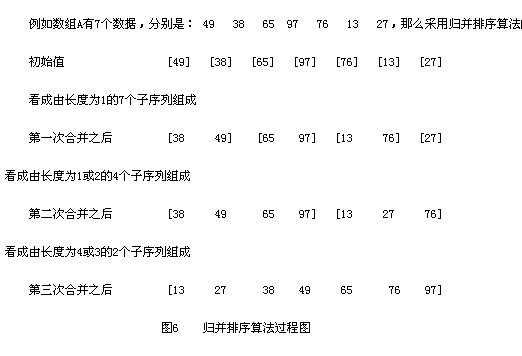``````public class MergeSort {
public void mergeSort(int[] arr) {
if (arr == null || arr.length == 0) {
return;
}
//辅助数组
int[] tmpArr = new int[arr.length];
mergeSort(arr, tmpArr, 0, arr.length - 1);
}

private void mergeSort(int[] arr, int[] tmpArr, int left, int right) {
if (left < right) {
int mid = (left + right) >> 1;
mergeSort(arr, tmpArr, left, mid);
mergeSort(arr, tmpArr, mid + 1, right);
merge(arr, tmpArr, left, mid, right);
}
}

private void merge(int[] arr, int[] tmpArr, int left, int mid, int right) {
int i = left;
int j = mid + 1;
int tmpIndex = left;
while (i <= mid && j <= right) {
if (arr[i] < arr[j]) {
tmpArr[tmpIndex++] = arr[i];
i++;
} else {
tmpArr[tmpIndex++] = arr[j];
j++;
}
}

while (i <= mid) {
tmpArr[tmpIndex++] = arr[i++];
}

while (j <= right) {
tmpArr[tmpIndex++] = arr[j++];
}

for (int m = left; m <= right; m++) {
arr[m] = tmpArr[m];
}
}
}
``````

### # 堆排序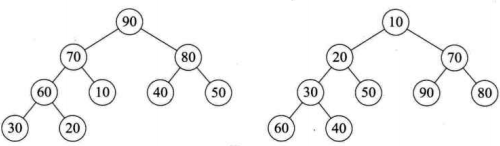Top大问题解决思路：使用一个固定大小的最小堆，当堆满后，每次添加数据的时候与堆顶元素比较，若小于堆顶元素，则舍弃，若大于堆顶元素，则删除堆顶元素，添加新增元素，对堆进行重新排序。

PriorityQueue 是一种基于优先级堆的优先级队列。每次从队列中取出的是具有最高优先权的元素。如果不提供Comparator的话，优先队列中元素默认按自然顺序排列，也就是数字默认是小的在队列头。优先级队列用数组实现，但是数组大小可以动态增加，容量无限。

``````//找出前k个最大数，采用小顶堆实现
public static int[] findKMax(int[] nums, int k) {
PriorityQueue<Integer> pq = new PriorityQueue<>(k);//队列默认自然顺序排列，小顶堆，不必重写compare

for (int num : nums) {
if (pq.size() < k) {
pq.offer(num);
} else if (pq.peek() < num) {//如果堆顶元素 < 新数，则删除堆顶，加入新数入堆
pq.poll();
pq.offer(num);
}
}

int[] result = new int[k];
for (int i = 0; i < k&&!pq.isEmpty(); i++) {
result[i] = pq.poll();
}
return result;
}
``````

## # 动态规划

### # 最长回文子串

1. 定义状态。`dp[i][j]` 表示子串 `s[i..j]` 是否为回文子串

2. 状态转移方程：`dp[i][j] = (s[i] == s[j]) and dp[i + 1][j - 1]`

3. 初始化的时候，单个字符一定是回文串，因此把对角线先初始化为 `true`，即 `dp[i][i] = true`

4. 只要一得到 `dp[i][j] = true`，就记录子串的长度和起始位置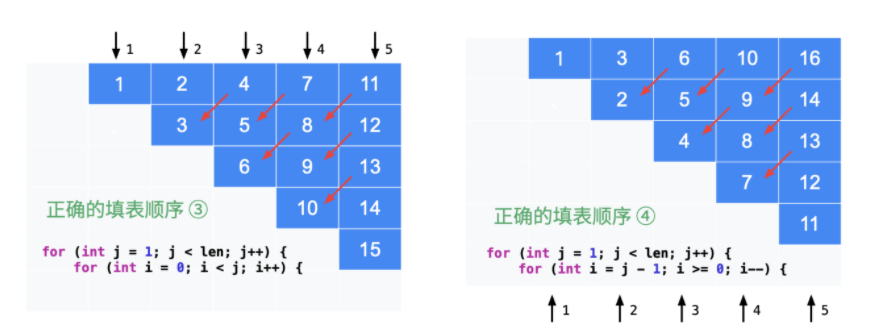``````public class Solution {

public String longestPalindrome(String s) {
// 特判
int len = s.length();
if (len < 2) {
return s;
}

int maxLen = 1;
int begin = 0;

// dp[i][j] 表示 s[i, j] 是否是回文串
boolean[][] dp = new boolean[len][len];
char[] charArray = s.toCharArray();

for (int i = 0; i < len; i++) {
dp[i][i] = true;
}
for (int j = 1; j < len; j++) {
for (int i = 0; i < j; i++) {
if (charArray[i] != charArray[j]) {
dp[i][j] = false;
} else {
if (j - i < 3) {
dp[i][j] = true;
} else {
dp[i][j] = dp[i + 1][j - 1];
}
}

// 只要 dp[i][j] == true 成立，就表示子串 s[i..j] 是回文，此时记录回文长度和起始位置
if (dp[i][j] && j - i + 1 > maxLen) {
maxLen = j - i + 1;
begin = i;
}
}
}
return s.substring(begin, begin + maxLen); //substring(i, j)截取i到j(不包含j)的字符串
}
}
``````

### # 最大子数组和

``````输入： nums = [-2,1,-3,4,-1,2,1,-5,4]

``````

1、首先确定dp数组（dp table）以及下标的含义。

dp[i]表示以nums[i]结尾的子数组的最大和。

2、确定递推公式。

`dp[i] = dp[i - 1] > 0 ? ( dp[i - 1] + nums[i]) : nums[i]`

dp[i+1]取决于dp[i]的值，不需要使用数组保存状态，只需要一个变量sum来保存上一个状态即可。

3、dp数组如何初始化。

dp应该是多少呢？根据dp[i]的定义，很明显dp应为nums即dp = nums。

``````class Solution {
public int maxSubArray(int[] nums) {
if (nums == null || nums.length == 0) {
return 0;
}

int max = nums;
int sum = 0;

for (int num : nums) {
if (sum > 0) {
sum += num;
} else {
sum = num;
}
max = Math.max(max, sum);
}

return max;
}
}
``````

### # 最长公共子序列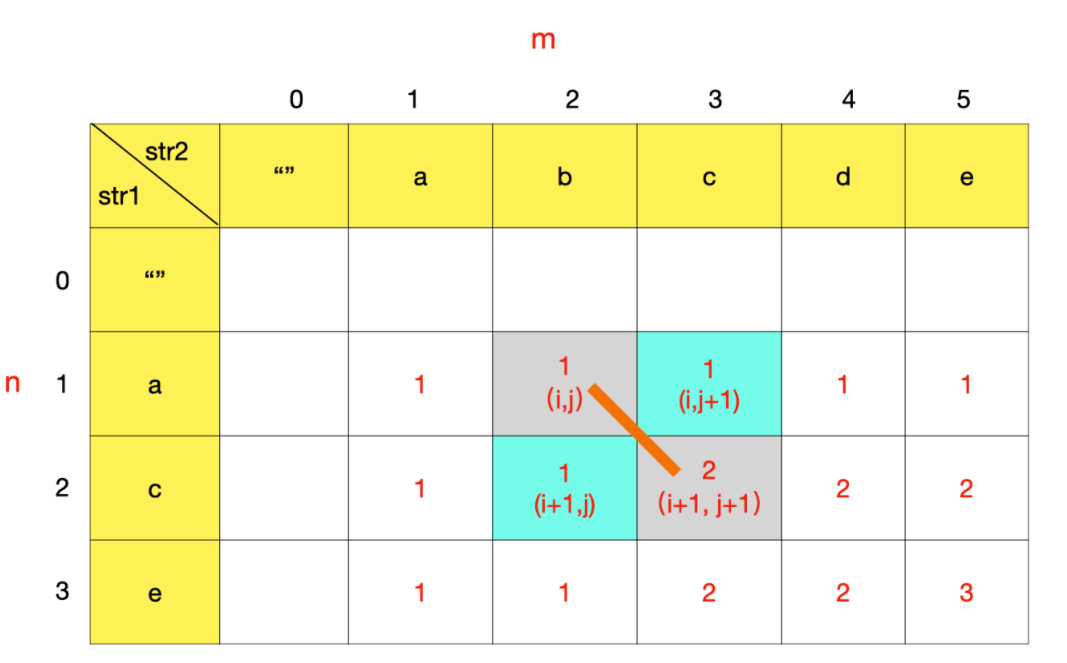``````class Solution {
public int longestCommonSubsequence(String text1, String text2) {
char[] arr1 = text1.toCharArray();
char[] arr2 = text2.toCharArray();
//dp[x]和dp[x]表示有一个为空字符串
//dp为text1第一个字符和text2第一个字符的最长公共子序列的长度
//dp[i][j]表示text1以i-1结尾的子串和text2以j-1结尾的子串的最长公共子序列的长度
int len1 = arr1.length;
int len2 = arr2.length;
int[][] dp = new int[len1 + 1][len2 + 1];

for (int i = 1; i < len1 + 1; i++) {
for (int j = 1; j < len2 + 1; j++) {
if (arr1[i - 1] == arr2[j - 1]) {
dp[i][j] = dp[i - 1][j - 1] + 1;
} else {
dp[i][j] = Math.max(dp[i - 1][j], dp[i][j - 1]);
}
}
}

return dp[len1][len2];
}
}
``````

`dp[i][j]`表示text1以i结尾的子串和text2以j结尾的子串的最长公共子序列的长度。需要处理base case。

``````class Solution {
public int longestCommonSubsequence(String text1, String text2) {
char[] arr1 = text1.toCharArray();
char[] arr2 = text2.toCharArray();

int len1 = arr1.length;
int len2 = arr2.length;
//`dp[i][j]`表示text1以i结尾的子串和text2以j结尾的子串的最长公共子序列的长度。
int[][] dp = new int[len1][len2];

if (arr1 == arr2) {
dp = 1;
}
for (int i = 1; i < len1; i++) {
if (arr1[i] == arr2) {
dp[i] = 1;
} else {
dp[i] = dp[i - 1];
}
}
for (int i = 1; i < len2; i++) {
if (arr1 == arr2[i]) {
dp[i] = 1;
} else {
dp[i] = dp[i - 1];
}
}

for (int i = 1; i < len1; i++) {
for (int j = 1; j < len2; j++) {
if (arr1[i] == arr2[j]) {
dp[i][j] = dp[i - 1][j - 1] + 1;
} else {
dp[i][j] = Math.max(dp[i - 1][j], dp[i][j - 1]);
}
}
}

return dp[len1 - 1][len2 - 1];
}
}
``````

### # 接雨水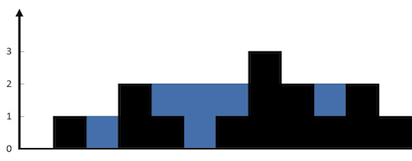``````输入：height = [0,1,0,2,1,0,1,3,2,1,2,1]

``````

``````class Solution {
public int trap(int[] height) {
int len = height.length;
int res = 0;
int[] leftMax = new int[len];
int[] rightMax = new int[len];

for (int i = 1; i < len; i++) {
leftMax[i] = Math.max(leftMax[i - 1], height[i - 1]);
}

for (int j = len - 2; j > 0; j--) {
rightMax[j] = Math.max(rightMax[j + 1], height[j + 1]);
}

for (int i = 1; i < len - 1; i++) {
int min = Math.min(leftMax[i], rightMax[i]);
if (min > height[i]) {
res += min - height[i];
}
}

return res;
}
}
``````

### # 单词拆分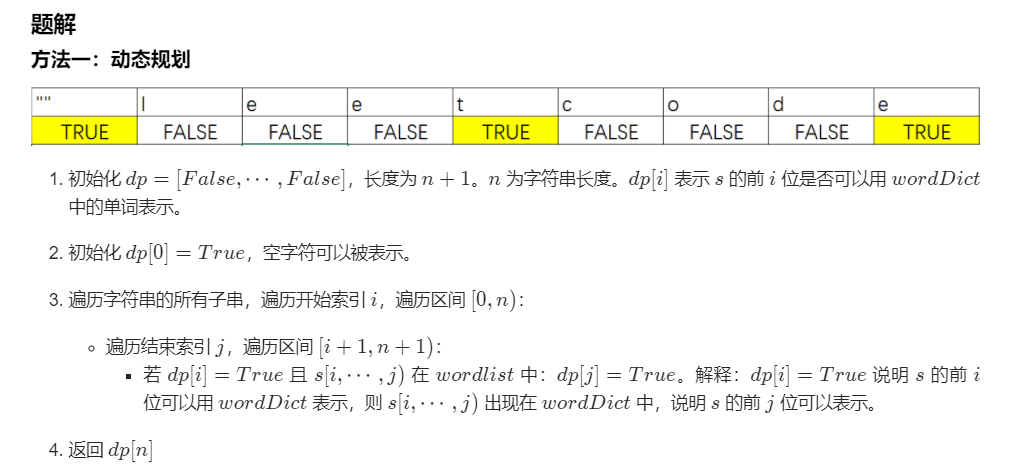``````class Solution {
public boolean wordBreak(String s, List<String> wordDict) {
int len = s.length(), maxw = 0;
//dp[i]表示前i个字母组成的字符串是否可以被拆分
boolean[] dp = new boolean[len + 1];
//状态转移方程初始化条件
dp = true;
Set<String> set = new HashSet();
for(String str : wordDict){
maxw = Math.max(maxw, str.length());
}
for(int i = 1; i < len + 1; i++){
for(int j = i; j >= 0 && j >= i - maxw; j--){
if(dp[j] && set.contains(s.substring(j, i))){
dp[i] = true;
break;
}
}
}
return dp[len];
}
}
``````

## # 回溯算法

### # 组合总和

``````输入：candidates = [2,3,6,7], target = 7,

[
,
[2,2,3]
]
``````

``````class Solution {
private List<List<Integer>> ans = new ArrayList<>();
public List<List<Integer>> combinationSum2(int[] candidates, int target) {
if (candidates == null || candidates.length == 0) {
return ans;
}
Arrays.sort(candidates);//排序方便回溯剪枝
combinationSum2Helper(candidates, target, 0, path);
return ans;
}

public void combinationSum2Helper(int[] arr, int target, int start, Deque<Integer> path) {
if (target == 0) {
}

for (int i = start; i < arr.length; i++) {
if (target < arr[i]) {//剪枝
return;
}
if (i > start && arr[i] == arr[i - 1]) {//在一个层级，会产生重复
continue;
}
combinationSum2Helper(arr, target - arr[i], i + 1, path);
path.removeLast();
}
}
}
``````

### # 全排列

``````输入: [1,2,3]

[
[1,2,3],
[1,3,2],
[2,1,3],
[2,3,1],
[3,1,2],
[3,2,1]
]
``````

``````class Solution {
private List<List<Integer>> ans = new ArrayList<>();
public List<List<Integer>> permute(int[] nums) {
boolean[] flag = new boolean[nums.length];
ArrayDeque<Integer> path = new ArrayDeque<>();
permuteHelper(nums, flag, path);

return ans;
}

private void permuteHelper(int[] nums, boolean[] flag, ArrayDeque<Integer> path) {
if (path.size() == nums.length) {
return;
}
for (int i = 0; i < nums.length; i++) {
if (flag[i]) {
continue;//继续循环
}
flag[i] = true;
permuteHelper(nums, flag, path);
path.removeLast();
flag[i] = false;
}
}
}
``````

### # 全排列II

1、排序；2、同一层级相同元素剪枝。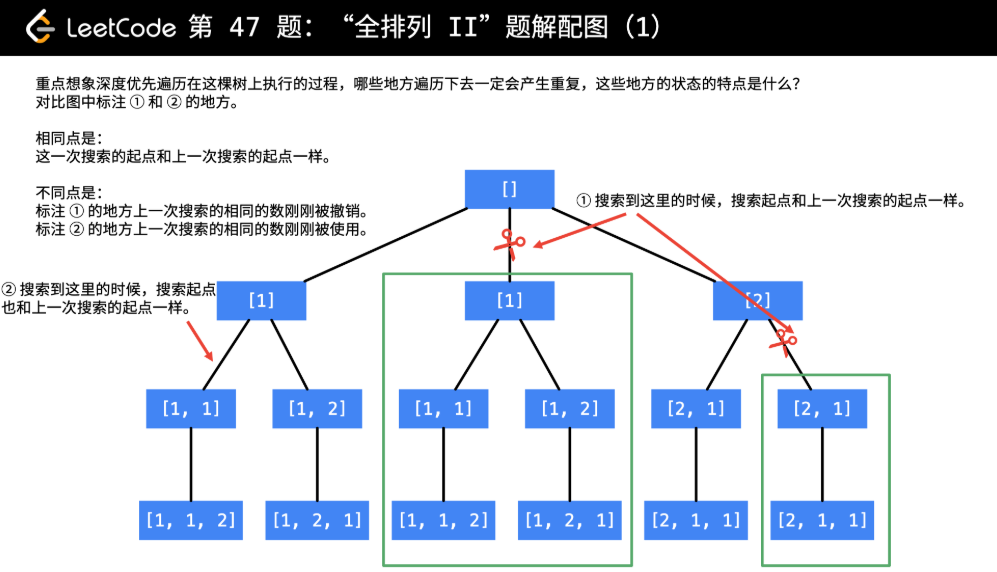``````class Solution {
private List<List<Integer>> ans = new ArrayList<>();
public List<List<Integer>> permuteUnique(int[] nums) {
if (nums == null || nums.length == 0) {
return ans;
}
ArrayDeque<Integer> path = new ArrayDeque<>();
boolean[] used = new boolean[nums.length];
Arrays.sort(nums);//切记
dps(nums, used, path);

return ans;
}

private void dps(int[] nums, boolean[] used, ArrayDeque<Integer> path) {
if (path.size() == nums.length) {
return;
}
for (int i = 0; i < nums.length; i++) {
if (used[i]) {
continue;
}
if ((i > 0 && nums[i] == nums[i - 1]) && !used[i - 1]) {//同一层相同的元素，剪枝
continue;//继续循环，不是return退出循环
}
used[i] = true;
dps(nums, used, path);
path.removeLast();
used[i] = false;
}
}
}
``````

## # 贪心算法

1. 从某个初始解出发；
2. 采用迭代的过程，当可以向目标前进一步时，就根据局部最优策略，得到一部分解，缩小问题规模；
3. 将所有解综合起来。

### # 买卖股票的最佳时机 II

``````输入：prices = [1,2,3,4,5]

总利润为 4 。
``````

``````//输入: [7,1,5,3,6,4]
//输出: 7
class Solution {
public int maxProfit(int[] prices) {
int profit = 0;
for (int i = 1; i < prices.length; i++) {
int tmp = prices[i] - prices[i - 1];
if (tmp > 0) {
profit += tmp;
}
}

return profit;
}
}
``````

### # 跳跃游戏

``````输入：nums = [2,3,1,1,4]

``````

1. 如果某一个作为 起跳点 的格子可以跳跃的距离是 3，那么表示后面 3 个格子都可以作为 起跳点
2. 可以对每一个能作为 起跳点 的格子都尝试跳一次，把 能跳到最远的距离 不断更新
3. 如果可以一直跳到最后，就成功了
``````class Solution {
public boolean canJump(int[] nums) {
if (nums == null || nums.length == 0) {
return true;
}

int maxIndex = nums;
for (int i = 1; i < nums.length; i++) {
if (maxIndex >= i) {
maxIndex = Math.max(maxIndex, i + nums[i]);
} else {
return false;
}
}

return true;
}
}
``````

### # 加油站

``````输入: gas = [1,2,3,4,5], cost = [3,4,5,1,2]

``````

1. 遍历一周，总获得的油量少于要花掉的油量必然没有结果；
2. 先苦后甜，记录遍历时所存的油量最少的站点，由于题目有解只有唯一解，所以从当前站点的下一个站点开始是唯一可能成功开完全程的。
``````class Solution {
public int canCompleteCircuit(int[] gas, int[] cost) {
int minIdx=0;
int sum=Integer.MAX_VALUE;
int num=0;
for (int i = 0; i < gas.length; i++) {
num+=gas[i]-cost[i];
if(num<sum){
sum=num;
minIdx=i;
}
}
return num<0?-1:(minIdx+1)%gas.length;
}
}
``````

## # 双指针

### # 反转链表

``````输入：head = [1,2,3,4,5]

``````

1. 定义两个指针，第一个指针叫 pre，最初是指向 null 的。
2. 第二个指针 cur 指向 head，然后不断遍历 cur。
3. 每次迭代到 cur，都将 cur 的 next 指向 pre，然后 pre 和 cur 前进一位。
4. 都迭代完了(cur 变成 null 了)，pre 就是最后一个节点了。
``````class Solution {
return null;
}

ListNode pre = null;
ListNode tmp = null;
while (cur != null) {
tmp = cur.next;
cur.next = pre;
pre = cur;
cur = tmp;
}

return pre;
}
}
``````

### # 反转链表II

``````输入：head = [1,2,3,4,5], left = 2, right = 4

``````

``````class Solution {
public ListNode reverseBetween(ListNode head, int m, int n) {
ListNode dummy = new ListNode(0);
ListNode pre = dummy;
for (int i = 1; i < m; i++) {
pre = pre.next;
}
for (int i = m; i < n; i++) {
cur.next = pre.next;
pre.next = cur;
}

return dummy.next;
}
}
``````

### # 删除链表倒数第n个节点

``````输入：head = [1,2,3,4,5], n = 2

``````

``````class Solution {
public ListNode removeNthFromEnd(ListNode head, int n) {
ListNode tmp = new ListNode(0); //技巧

ListNode fast = tmp;
ListNode slow = tmp;

while (n-- > 0) {
fast = fast.next;
}

while (fast.next != null) {
fast = fast.next;
slow = slow.next;
}
slow.next = slow.next.next;

return tmp.next;
}
}
``````

### # 三数之和

``````输入：nums = [-1,0,1,2,-1,-4]

``````

• 首先对数组进行排序，排序后固定一个数 nums[i]nums[i]，再使用左右指针指向 nums[i]nums[i]后面的两端，数字分别为 nums[L]nums[L] 和
• nums[R]nums[R]，计算三个数的和 sumsum 判断是否满足为 00，满足则添加进结果集
• 如果 nums[i]nums[i]大于 00，则三数之和必然无法等于 00，结束循环
• 如果 nums[i]nums[i] == nums[i-1]nums[i−1]，则说明该数字重复，会导致结果重复，所以应该跳过
• 当 sumsum == 00 时，nums[L]nums[L] == nums[L+1]nums[L+1] 则会导致结果重复，应该跳过，L++L++
• 当 sumsum == 00 时，nums[R]nums[R] == nums[R-1]nums[R−1] 则会导致结果重复，应该跳过，R--R−−

``````class Solution {
public List<List<Integer>> threeSum(int[] nums) {
List<List<Integer>> res = new ArrayList<>();
Arrays.sort(nums);

for (int i = 0; i < nums.length; i++) {
if (nums[i] > 0) { //最左边的数字大于0，则sum不会等于0，退出
break;
}
if (i > 0 && nums[i] == nums[i - 1]) { //去重复
continue;
}

int left = i + 1;
int right = nums.length - 1;

while (left < right) {
int sum = nums[i] + nums[left] + nums[right];
if (sum == 0) {
res.add(Arrays.asList(nums[i], nums[left], nums[right])); ///array to list
while (left < right && nums[left] == nums[left + 1]) {
left++;
}
while (left < right && nums[right] == nums[right - 1]) {
right--;
}
left++;
right--;
} else if (sum > 0) {
right--;
} else {
left++;
}
}
}

return res;
}
}
``````

### # 环形链表

``````输入：head = [3,2,0,-4], pos = 1

``````

``````public class Solution {
return false;
}

while (quick != null && quick.next != null) {
slow = slow.next;
quick = quick.next.next;

if (slow == quick) {
return true;
}
}

return false;
}
}
``````

### # 环形链表II

``````输入：head = [3,2,0,-4], pos = 1

``````

``````public class Solution {

while (true) {
if (fast == null || fast.next == null) {
return null;
}
fast = fast.next.next;
slow = slow.next;
if (fast == slow) {
break;
}
}

while (slow != fast) {
slow = slow.next;
fast = fast.next;
}

return fast;
}
}
``````

``````public class Solution {
//快慢指针找出环的大小
while (fast != null && fast.next != null) {
slow = slow.next;
fast = fast.next.next;
if (fast == slow) {
break;
}
}

if (fast == null || fast.next == null) {
return null;
}

int cycleSize = 1;
while (fast.next != slow) {
cycleSize++;
fast = fast.next;
}

//快慢指针重新从链表首部出发，快指针先走sizeOfCycle步
//然后两个指针同时一起走，步长为1，相遇节点即是环的入口